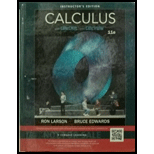Chapter 3.3, Problem 96E### Calculus (MindTap Course List)

11th Edition
Ron Larson + 1 other
ISBN: 9781337275347

#### Solutions

Chapter
Section### Calculus (MindTap Course List)

11th Edition
Ron Larson + 1 other
ISBN: 9781337275347
Textbook Problem

# True or False? In Exercises 91-96, determine whether the statement is true or false. If it is false, explain why or give an example that shows it is false.The relative maxima of the function f are f ( 1 ) = 4 and f ( 3 ) = 10 , Therefore, f has at least one minimum for some x in the interval (1, 3).

To determine
Whether the provided statement,“A function with two maximas f(1)=4 and f(3)=10 would have a minima in the interval (1,3)” is true or false.

Explanation

Given:

The statement “A function with two maximas f(1)=4 and f(3)=10 would have a minima in the interval (1,3).

Explanation:

According to the first derivative test, for a critical point c of a function f(x),

If f'(x) changes sign from a negative value to a positive value at the point c, the f is said to have a relative minima at the point c.

If f'(x) changes sign from a positive value to a negative value at the point c, the f is said to have a relative maxima at the point c

### Still sussing out bartleby?

Check out a sample textbook solution.

See a sample solution

#### The Solution to Your Study Problems

Bartleby provides explanations to thousands of textbook problems written by our experts, many with advanced degrees!

Get Started

## Additional Math Solutions

#### Evaluate the definite integral. 01dx(1+x)4

Single Variable Calculus: Early Transcendentals, Volume I

#### Solve the equations in Exercises 126. 2x3x2x3x+1=0

Finite Mathematics and Applied Calculus (MindTap Course List)

#### In Problems 7-34, perform the indicated operations and simplify. 22.

Mathematical Applications for the Management, Life, and Social Sciences

#### Prove Property 3 of integrals.

Single Variable Calculus: Early Transcendentals

#### Subtract the following numbers. 23. $127 less$33

Contemporary Mathematics for Business & Consumers